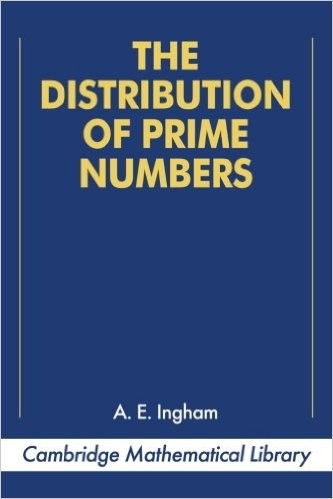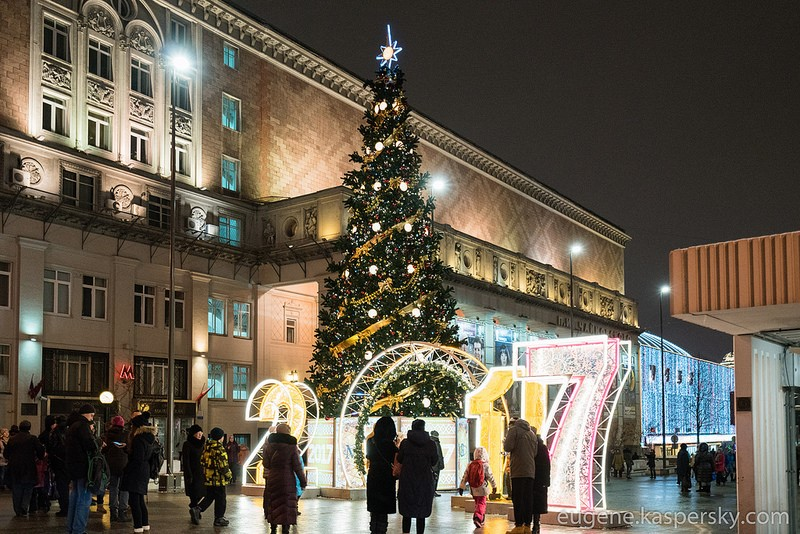# 2017: Prime Numbers, Factorials, Primorials, Derangements: It’s Complicated.

As many will already know, the number 2017 is a prime number; that is, it can be divided without a remainder only by itself and 1. Must say, the theory of prime numbers is a wholly interesting one and an extremely useful one too, as any cryptographer will tell you :).But today I’ll be writing about something different. See, based on the fact that 2017 is prime – or ‘simple’ – many, myself included, are anticipating a simple, straightforward and calm year 2017, especially since 2016 was a bit of a rotter. Let me show you why.

Like I said, prime numbers are those that can only be divided by themselves and 1 without leaving a remainder. Non-prime numbers are called composite numbers, incidentally.

Turns out that 2016 is not only a composite number but a very composite number! It has a whole eight divisors. Grab a calculator your smartphone and test it for yourself:
2016 = 2 * 2 * 2 * 2 * 2 * 3 * 3 * 7

Whoah! Even the quantity of divisors is anything but simple, since 8 = 2 * 2 * 2.

So what about other years? Was 1917, the year of the Russian Revolution, a ‘prime’ year, for example? No, it wasn’t. 1917 = 3 * 3 * 3 * 71. Just four divisors, but they’re kinda poignant – and prophetic of nothing much good.

So what about other very prime/simple years, and other very non-prime/non-simple ones? Ok, let’s narrow this down a bit to 1980 through present day…

Prime/simple years:
1987
1993
1997
1999
2003
2011

And in the near future there are a few more prime/simples:
2027
2029

(eek, that’s a lot of non-simple years until then)

The most non-prime/non-simple years were:
1984 = 2 * 2 * 2 * 2 * 2 * 2 * 31 (seven divisors)
2000 = 2 * 2 * 2 * 2 * 5 * 5 * 5   (also seven)

There were six divisors in 1980, and there’ll be six in 2025. All other years can be called semi-prime/semi-simple.

But I digress…

Now, in the popular British mathematical journal The Guardian :), readers were recently teased with a… brain teaser. In the blanks between the sequence of figures 10 9 8 7 6 5 4 3 2 1 you need to add arithmetic symbols (+, -, x, ÷, (),) – as many as you like – so as to get the number (year) 2017.For example, if you add arithmetic signs as follows you get 817:
10 * 9 * (8 + 7 – 6) * (5 – 4) + 3 * 2 + 1 = 817

But how do you add arithmetic to get 2017?
10?9?8?7?6?5?4?3?2?1 = 2017

Come on, have a go!

As for me, in nine minutes I got the equation to equal 2017 by kinda wonky arithmetic (I made the ‘3’ and ‘2’ = ’32’!); then, in around 15 or 20 minutes I got the answer in a proper way without bending the rules. I say ‘a’ way: there are different ways of getting to 2017!

So, tried it yet?

Ok, let’s make it harder: Now take away the 10:
9  8  7  6  5  4  3  2  1 = 2017

Hmmm. I say harder; I got 2017 in two minutes. I guess I was already more experienced ).

Ok, now really harder:
8  7  6  5  4  3  2  1 = 2017

7  6  5  4  3  2  1 = 2017
and
6  5  4  3  2  1 = 2017

I found I needed to add the factorial for these two.

To summarize:
10  9  8  7  6  5  4  3  2  1 = 2017
9  8  7  6  5  4  3  2  1 = 2017
8  7  6  5  4  3  2  1 = 2017

(using +, -, x, ÷, () )

And with the factorial (n!):
7  6  5  4  3  2  1 = 2017
6  5  4  3  2  1 = 2017

Because you need extra math symbols, basic ‘+ – *’ aren’t enough:

7*6*5*4*3 = 2520, to get 2017 you have to make 503 out of ‘2 and 1″. Not possible. The rest of calculation variants result in numbers smaller than 2017. So, let’s have the factorial.

5 4 3 2 1
4 3 2 1

Of course, the task is more complicated and one needs more math:

multifactorials, primorials, superfactorials, derangements, and the square root if you like.

Not so bored with the math? Ok, here you go:
3 2 1
2 1

Do you think it’s impossible? The math is wide and deep, with more surprises for inexperienced junior calculators (in the old English meaning). Read about it here.

Thus, everything is possible. I’m not kidding. Let’s do 2017 out of… just ‘1’!

The most elegant, witty, simple (etc.) answer will get a license for the best Internet Security in the world!

So, three-two-one – go!#### M.Venkatesan

Hi Eugene, the following link provides representation of numbers 0 to 11111 in terms of increasing and decreasing orders of 1 to 9.

https://arxiv.org/pdf/1302.1479v5.pdf#### Apratim Mukherjee

32*7*8+(4*5+(6-1))*9= 2017#### Apratim Mukherjee

2(6-4-5-3)17= 2017

Leave a note